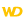WINDEV, WEBDEV AND WINDEV MOBILEONLINE HELPVersion: 15161718192021222324
 Home |Sign in | EnglishHelpWLanguageWLanguage functionsControls, pages and windowsDrawing functions (Image controls and variables)Object syntaxFunctions for drawing on Image variables (object syntax)DrawRectangleGradientPresentationExampleGradient parametersRelated examplesSee alsoAlphaBlendBackgroundChangeModeCopyCropDominantImageColorDrawArcDrawBorderDrawChordDrawCircleDrawHaloDrawLineDrawPointDrawPolygonDrawPolylineDrawRectangleDrawRectangleGradientDrawRoundedRectangleDrawShadowDrawSliceDrawTextDrawTextRTFEncrustColorEndDrawingFillFontHorizontalSymmetryInvertColorModifyHSLModifyHueModifyLightnessModifySaturationPenPixelColorPixelOpacityResizeRotationSaveBMPSaveGIFSaveJPEGSavePNGStartDrawingVerticalSymmetryNew WINDEV, WEBDEV, WINDEV Mobile 24 feature!
• Gradient parameters
WINDEVWEBDEVWINDEV MobileOthers<Image variable>.DrawRectangleGradient (Function)
In french: <Variable Image>.DessineRectangleDégradé
Draws a rectangle in an Image variable.
Example
MyImage is Image = "Test.gif"
// Draw a rectangle whose background changes from red to blue
// via yellow
MyImage.DrawRectangleGradient(10, 20, 100, 150, LightRed, LightBlue, 0, LightYellow, 30)
IMG_MyDrawing = MyImage

Syntax

Drawing a rectangle by specifying the points of the rectangle and the gradient

<Image variable>.DrawRectangleGradient(<X1> , <Y1> , <X2> , <Y2> , <Start color> , <End color> [, <Angle> [, <Color 3> [, <Distance color 3> [, <Color 4> [, <Distance color 4>]]]]])
<Image variable>: Image variable
Name of Image variable to use.
<X1>: Integer
X coordinate (in pixels) of top left corner of rectangle.
<Y1>: Integer
Y coordinate (in pixels) of top left corner of rectangle.
<X2>: Integer
X coordinate (in pixels) of bottom right corner of rectangle.
<Y2>: Integer
Y coordinate (in pixels) of bottom right corner of rectangle.
<Start color>: Integer or constant
Start color of gradient. This color corresponds to:
<End color>: Integer or constant
End color of gradient. This color corresponds to:
<Angle>: Optional integer
Angle that will be used for the selection of colors in the gradient. The angle value is included between 0 (horizontal angle, default value) and 360°.
<Color 3>: Optional constant or integer
Color that will be used in the gradient. This color corresponds to:
<Distance color 3>: Optional integer
Percentage separating the start color from color 3.
<Color 4>: Optional constant or integer
Color that will be used in the gradient. This color corresponds to:
<Distance color 4>: Optional integer
Percentage separating the start color from color 4.Drawing a rectangle by specifying the points of the rectangle and by using the Background type

<Image variable>.DrawRectangleGradient(<X1> , <Y1> , <X2> , <Y2> , <Gradient>)
<Image variable>: Image variable
Name of Image variable to use.
<X1>: Integer
X coordinate (in pixels) of top left corner of rectangle.
<Y1>: Integer
Y coordinate (in pixels) of top left corner of rectangle.
<X2>: Integer
X coordinate (in pixels) of bottom right corner of rectangle.
<Y2>: Integer
Y coordinate (in pixels) of bottom right corner of rectangle.
<Gradient>: Background variable
Name of the Background variable that contains the characteristics of the gradient.Drawing a rectangle by using the Rectangle type and by specifying the gradient

<Image variable>.DrawRectangleGradient(<Rectangle> , <Start color> , <End color> [, <Angle> [, <Color 3> [, <Distance color 3> [, <Color 4> [, <Distance color 4>]]]]])
<Image variable>: Image variable
Name of the Image variable to use.
<Rectangle>: Rectangle variable
Name of Rectangle variable that defines the coordinates of the rectangle to draw.
<Start color>: Integer or constant
Start color of gradient. This color corresponds to:
<End color>: Integer or constant
End color of gradient. This color corresponds to:
<Angle>: Optional integer
Angle that will be used for the selection of colors in the gradient. The angle value is included between 0 (horizontal angle, default value) and 360°.
<Color 3>: Optional constant or integer
Color that will be used in the gradient. This color corresponds to:
<Distance color 3>: Optional integer
Percentage separating the start color from color 3.
<Color 4>: Optional constant or integer
Color that will be used in the gradient. This color corresponds to:
<Distance color 4>: Optional integer
Percentage separating the start color from color 4.Versions 21 and laterDrawing a rectangle by using the Rectangle type and the Background type

<Image variable>.DrawRectangleGradient(<Rectangle> , <Gradient>)
<Image variable>: Image variable
Name of the Image variable to use.
<Rectangle>: Rectangle variable
Name of Rectangle variable that defines the coordinates of the rectangle to draw.
<Gradient>: Background variable
Name of Background variable containing the characteristics of gradient.New in version 21Drawing a rectangle by using the Rectangle type and the Background type

<Image variable>.DrawRectangleGradient(<Rectangle> , <Gradient>)
<Image variable>: Image variable
Name of the Image variable to use.
<Rectangle>: Rectangle variable
Name of Rectangle variable that defines the coordinates of the rectangle to draw.
<Gradient>: Background variable
Name of Background variable containing the characteristics of gradient.Drawing a rectangle by using the Rectangle type and the Background type

<Image variable>.DrawRectangleGradient(<Rectangle> , <Gradient>)
<Image variable>: Image variable
Name of the Image variable to use.
<Rectangle>: Rectangle variable
Name of Rectangle variable that defines the coordinates of the rectangle to draw.
<Gradient>: Background variable
Name of Background variable containing the characteristics of gradient.
Remarks

Gradient parameters

The color parameters are used as follows:The angle is used to define the orientation of the gradient.The gradients performed with 3 or 4 colors are only available for an angle set to 0 or 90 degrees.
Note: The gradient colors are not available for some systems (Windows 98 or Me for example) or for TSE 256 colors. In this case, a rectangle whose color corresponds to <Start color> is displayed.
Related Examples:Unit examples (WINDEV): The drawing functions [ + ] Using the main drawing functions of WINDEV to:- Initialize an Image control for drawing- Draw simple shapes- Write a text into a drawing- Change the color in a drawing
Component : wd240pnt.dll
Minimum version required
• Version 24
This page is also available for…
 Comments
 Click [Add] to post a comment
 Registered trademarks. Copyright ©2009-2019 PC SOFT. All rights reservedThis website has been developed with WEBDEV and HFSQLWEBDEV, WINDEV Mobile and WINDEV programming